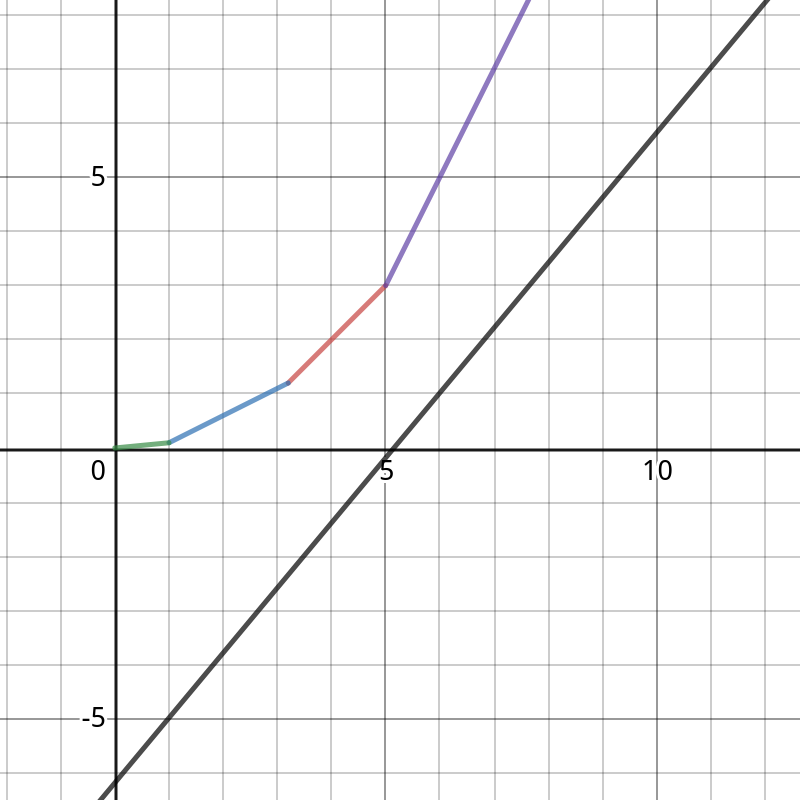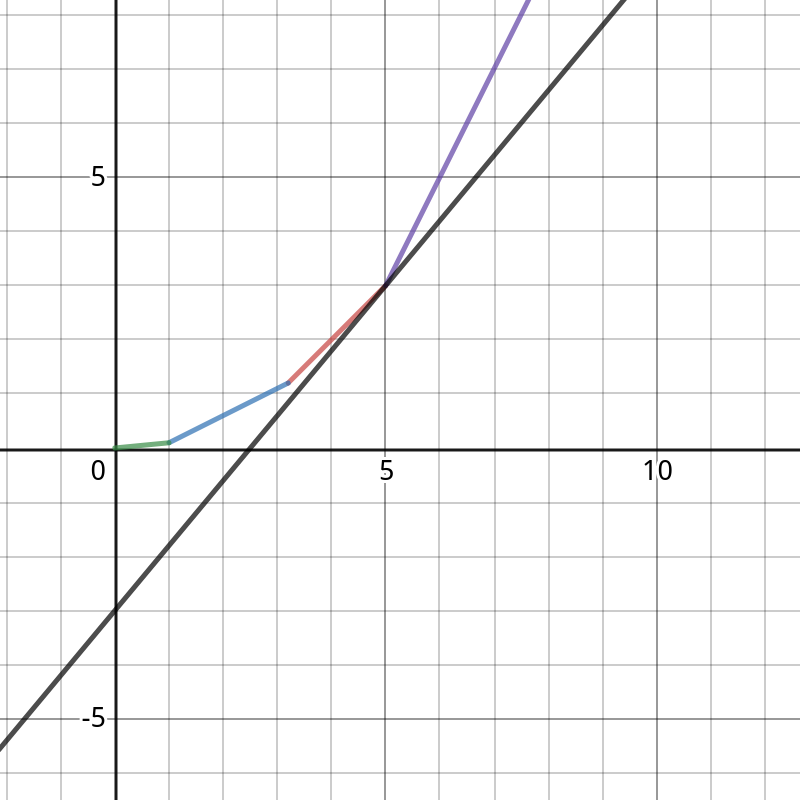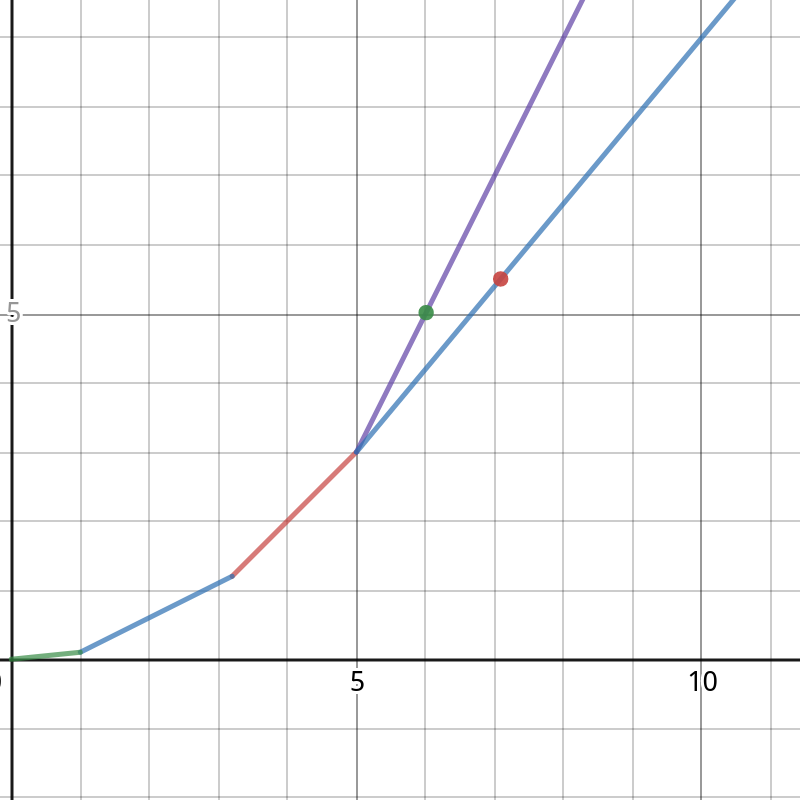# arrow_back动态规划共96篇文章zc
2019-12-21 19:47:00

f_i表示金额为i有多少种方案

f_i = \sum_{j=1}^4 f_{i-c[j](i \ge c_j)}

1超额

1,2超额

1,3超额

1,4超额

1,2,3超额

1,2,4超额

1,3,4超额

1,2,3,4超额

...

(1,2,3)(1,2,4)又是多算的...

(当有奇数种不合法的时候减去,偶数种不合法时加上)zc
2019-12-21 19:47:00

f[x]表示状态为x时最少走多少步

f[x|2^{i-1}|2^{j-1}]=\min(f[x|2^{i-1}|2^{j-1}],f[x])

(如果不懂可以自己模拟一下)zc
2019-12-21 19:47:00zc
2019-12-21 19:47:00

f_i表示前i件玩具的制作费用

 f_i=\min_{j=1}^{i-1}(f[j]+(i-j-1+s_i-s_j-L)^2) 

a_i=s_i+i,b[i]=s_i+i+L+1

(都只与i有关)

x=b_j,y=f_j+{b_j}^2  y=2a_ix+(f_i-{a_i}^2) 

f_i含义转化为当上述直线经过点(x,y)(b_j,f_j+{b_j}^2)时,直线在y轴的截距上加上{a_i}^2

https://www.desmos.com/calculator/mqsdvzuaee1. while(s(q_h,q_{h+1})<2a_i)++h;
2. 当前队头的点最优,用这个点计算出f_i
3. while(s(q_{t-1},q_t)>s(q_{t-1},i))--t;
4. 队尾插入点izc
2019-12-21 19:47:00

Easy top: 0

Solution： 　　期望题总是贼有意思。

本题期望comboo的期望连续长度的平方,所以我们设f_i表示到了第i位的总期望combo,g_i表示到了第i位结尾的连续o的期望长度,那么分情况讨论：

1. s_i == o
2. s_i == x
3. s_i ==\ ?zc
2019-12-21 19:47:00zc
2019-12-21 19:47:00

f_{k,i}:第i位,改变k

res=MAX(a_i,..,a_j)-\sum_{t=j-1}^i a_tzc
2019-12-21 19:47:00zc
2019-12-21 19:47:00

考虑 up:

s_x为点x子树中所有点到x的距离之和

S_x = \sum (s_{to}+siz_{to})

考虑 down:

s_{to} = s_{to} + (s_x-s_{to}-siz_{to}) + n - siz_{to}zc
2019-12-21 19:47:00

f[i][j]表示第i天有j只股票最多赚多少钱 \\

1. 直接买 f[i][j] = -ap[i] \times j
2. 不买不卖

f[i][j] =f[i-1][j]

3. 买股

f[i][j] = f[i-w-1][k] - ap[i] \times (j-k) (j-as[i] \le k \le j)

4. 卖股

f[i][j] = f[i-w-1][k] + bp[i] \times (k-j) (j \le k \le j + bs[i])

Search
search